Home
Hostname: page-component-544b6db54f-vq995 Total loading time: 0.165 Render date: 2021-10-18T06:01:47.815Z Has data issue: true Feature Flags: { "shouldUseShareProductTool": true, "shouldUseHypothesis": true, "isUnsiloEnabled": true, "metricsAbstractViews": false, "figures": true, "newCiteModal": false, "newCitedByModal": true, "newEcommerce": true, "newUsageEvents": true }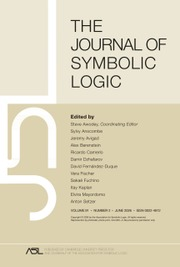The Journal of Symbolic Logic

# DEFINABLE MINIMAL COLLAPSE FUNCTIONS AT ARBITRARY PROJECTIVE LEVELS

Published online by Cambridge University Press:  14 March 2019

## Abstract

Using a nonLaver modification of Uri Abraham’s minimal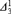$\Delta _3^1$ collapse function, we define a generic extension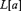$L[a]$ by a real a, in which, for a given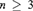$n \ge 3$,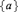$\left\{ a \right\}$ is a lightface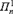$\Pi _n^1$ singleton, a effectively codes a cofinal map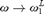$\omega \to \omega _1^L$ minimal over L, while every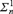$\Sigma _n^1$ set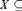$X \subseteq \omega$ is still constructible.

## Keywords

Type
Articles
Information
The Journal of Symbolic Logic , March 2019 , pp. 266 - 289

## Access options

Get access to the full version of this content by using one of the access options below. (Log in options will check for institutional or personal access. Content may require purchase if you do not have access.)

## References

Abraham, U., A minimal model for$\neg \,CH:$ Iteration of Jensen’s reals. Transactions of the American Mathematical Society , vol. 281 (1984), pp. 657674.Google Scholar
Abraham, U., Minimal model of “$\aleph _1^L$ is countable” and definable reals. Advances in Mathematics, vol. 55 (1985), pp. 7589.Google Scholar
Barwise, J. (ed.), Handbook of Mathematical Logic, Studies in Logic and the Foundations of Mathematics, vol. 90, North-Holland, Amsterdam, 1977.10.1016/S0049-237X(08)71097-8CrossRefGoogle Scholar
Bukovsky, L., Changing cofinality of$\aleph _2$, Set Theory and Hierarchy Theory, Memorial Tribute A. Mostowski (Marek, W., Srebrny, M., and Zarach, A., editors), Lecture Notes in Mathematics, vol. 537, Springer-Verlag, Berlin, 1976, pp. 3749.10.1007/BFb0096892CrossRefGoogle Scholar
Bukovský, L. and Copláková-Hartová, E., Minimal collapsing extensions of models of ZFC. Annals of Pure and Applied Logic, vol. 46 (1990), no. 3, pp. 265298.Google Scholar
Cummings, J., Iterated forcing and elementary embeddings, Handbook of Set Theory, vol. 3 (Foreman, M. and Kanamori, A., editors), Springer, Dordrecht, 2010, pp. 775883.10.1007/978-1-4020-5764-9_13CrossRefGoogle Scholar
Fischer, V., Friedman, S. D., Mejía, D. A., and Montoya, D. C., Coherent systems of finite support iterations, this Journal, vol. 83 (2018), no. 1, pp. 208236.Google Scholar
Friedman, S.-D., Gitman, V., and Kanovei, V., A model of second-order arithmetic satisfying AC but not DC. Journal of Mathematical Logic, to appear, 2018, arXiv:1808.04732.Google Scholar
Golshani, M., Kanovei, V., and Lyubetsky, V., A Groszek – Laver pair of undistinguishable$E_0$ classes . Mathematical Logic Quarterly, vol. 63 (2017), no. 1–2, pp. 1931.Google Scholar
Harrington, L., Handwritten notes, in four parts: (A) The constructible reals can be anything. May 1974.(B) Addendum. Models where Separation principles fail. May 1974. (C) Separation without Reduction. April 1975. (D) The constructible reals can be (almost) anything. Part II. May 1975.Google Scholar
Jech, T., Set Theory, The third millennium revised and expanded edition, Springer-Verlag, Berlin-Heidelberg, New York, 2003.Google Scholar
Jensen, R. B. and Solovay, R. M., Some applications of almost disjoint sets, Mathematical Logic and Foundations of Set Theory Proceedings of an International Colloquium, Jerusalem 1968 (Bar-Hillel, Y., editor), North-Holland, Amsterdam-London, 1970, pp. 84104.Google Scholar
Jensen, R., Definable sets of minimal degree, Mathematical Logic and Foundations of Set Theory Proceedings of an International Colloquium, Jerusalem 1968 (Bar-Hillel, Y., editor), North-Holland, Amsterdam-London, 1970, pp. 122128.Google Scholar
Kanamori, A., Perfect-set forcing for uncountable cardinals. Annals of Mathematical Logic, vol. 19 (1980), pp. 97114.Google Scholar
Kanovei, V., On the nonemptiness of classes in axiomatic set theory. Mathematics of the USSR-Izvestiya, vol. 12 (1978), pp. 507535.Google Scholar
Kanovei, V., Non-Glimm-Effros equivalence relations at second projective level. Fundamenta Mathematicae, vol. 154 (1997), no. 1, pp. 135.Google Scholar
Kanovei, V. and Lyubetsky, V., A definable$E_0$-class containing no definable elements. Archive for Mathematical Logic, vol. 54 (2015), no. 5, pp. 711723.Google Scholar
Kanovei, V., Counterexamples to countable-section${\rm{\Pi }}_2^1$ uniformization and${\rm{\Pi }}_3^1$ separation. Annals of Pure and Applied Logic, vol. 167 (2016), no. 4, pp. 262283.Google Scholar
Kanovei, V., Definable$E_0$ classes at arbitrary projective levels. Annals of Pure and Applied Logic, vol. 169 (2018), no. 9, pp. 851871.Google Scholar
Kanovei, V., Non-uniformizable sets of second projective level with countable cross-sections in the form of Vitali classes. Izvestiya: Mathematics, vol. 82 (2018), no. 1, pp. 6596.Google Scholar
Kanovei, V., Non-uniformizable sets with countable cross-sections on a given level of the projective hierarchy. Fundamenta Mathematicae, to appear, (2018).Google Scholar
Kurilić, M. S., Changing cofinalities and collapsing cardinals in models of set theory. Annals of Pure and Applied Logic, vol. 120 (2003), no. 1–3, pp. 225236.Google Scholar
Namba, K., Independence proof of$\left( {\omega ,\omega _\alpha } \right)$-distributive law in complete Boolean algebras. Commentarii Mathematici Universitatis Sancti Pauli, vol. 19 (1971), pp. 112.Google Scholar
5
Cited by

# Send article to Kindle

Note you can select to send to either the @free.kindle.com or @kindle.com variations. ‘@free.kindle.com’ emails are free but can only be sent to your device when it is connected to wi-fi. ‘@kindle.com’ emails can be delivered even when you are not connected to wi-fi, but note that service fees apply.

Find out more about the Kindle Personal Document Service.

DEFINABLE MINIMAL COLLAPSE FUNCTIONS AT ARBITRARY PROJECTIVE LEVELS
Available formats
×

# Send article to Dropbox

To send this article to your Dropbox account, please select one or more formats and confirm that you agree to abide by our usage policies. If this is the first time you use this feature, you will be asked to authorise Cambridge Core to connect with your <service> account. Find out more about sending content to Dropbox.

DEFINABLE MINIMAL COLLAPSE FUNCTIONS AT ARBITRARY PROJECTIVE LEVELS
Available formats
×

# Send article to Google Drive

To send this article to your Google Drive account, please select one or more formats and confirm that you agree to abide by our usage policies. If this is the first time you use this feature, you will be asked to authorise Cambridge Core to connect with your <service> account. Find out more about sending content to Google Drive.

DEFINABLE MINIMAL COLLAPSE FUNCTIONS AT ARBITRARY PROJECTIVE LEVELS
Available formats
×
×